# Chapter 8: Statistics And Probability - Online Test

Q1. Which of the following is not a measure of dispersion?
Explaination / Solution:
No Explaination.

Q2. The range of the data 8, 8, 8, 8, 8 . . . 8 is
Explaination / Solution:

Range = L - S = 8 - 8 = 0
Q3. The sum of all deviations of the data from its mean is
Explaination / Solution: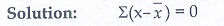Q4. The mean of 100 observations is 40 and their standard deviation is 3. The sum of squares of all deviations is
Explaination / Solution: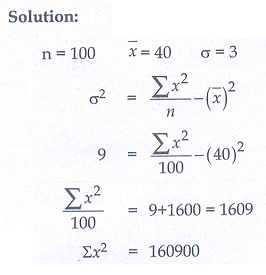Q5. Variance of first 20 natural numbers is
Explaination / Solution: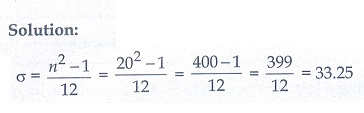Q6. The standard deviation of a data is 3. If each value is multiplied by 5 then the new variance is
Explaination / Solution: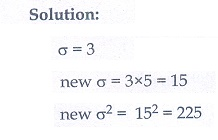Q7. If the standard deviation of x, y, z is p then the standard deviation of 3x + 5 , 3y + 5 , 3z + 5 is
Explaination / Solution:Q8. If the mean and coefficient of variation of a data are 4 and 87.5% then the standard deviation is
Explaination / Solution: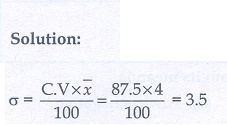Q9. Which of the following is incorrect?
Explaination / Solution:
No Explaination.

Q10. The probability a red marble selected at random from a jar containing p red, q blue and r green marbles is
Explaination / Solution: